# The Excel AND FunctionClaudia Buckley#### Join the Excel conversation on Slack

Ask a question or join the conversation for all things Excel on our Slack channel.

The Excel AND function is used to check whether the value in a cell meets multiple stated criteria. The AND function can be found under the logical functions in the Excel Function Library.

## Syntax

The syntax of the AND function is:

``=AND(logical1, [logical2],...)``

Up to 255 logical expressions can be evaluated, and if all stated conditions are true, the AND function returns a value of TRUE. If any stated condition is false, the return value is FALSE.

## How to use the AND function

Use this free Excel file to practice along with the tutorial.

Since it’s a logical function, the AND function is used with the standard logical operators >, <, = (greater than, less than, equal to, respectively). The following combinations of these operators are also used for even more specific comparisons.

Operator

Meaning

>=

Greater than or equal to

<=

Less than or equal to

<>

Not equal to

The AND function evaluates one cell at a time, so A1>500 is a valid logical statement within Excel, but A1:A4>500 is not because A1:A4 represents a range of cells. Statements that are unable to be evaluated will return a #VALUE! result, as shown below.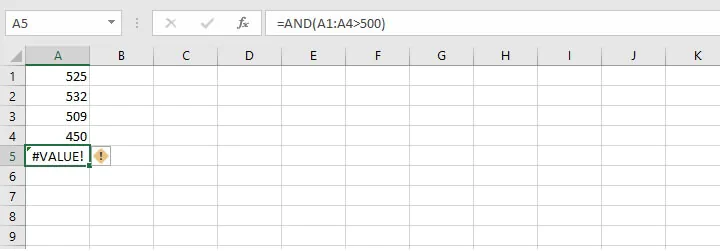In order to determine whether each cell within the range A2 to A4 is greater than 500, this formula would be used as shown in the image below.

``=AND(A1>500, A2>500, A3>500, A4>500)``

Excel returns a value of FALSE because the statement A4>500 is false.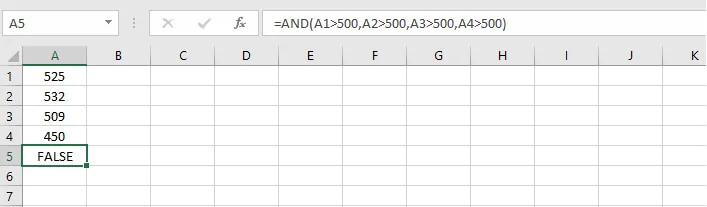If the value in cell A4 is changed to 550, we would get a TRUE result.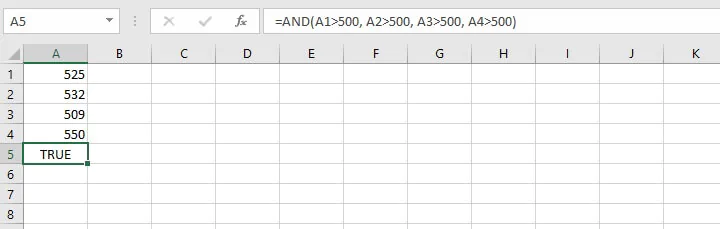### Example 1

In the following practical example, a bank will approve loans for customers with a minimum annual income of \$35,000 and a credit rating of at least 650. A customer who fails either of those criteria will not be approved for the loan. The following customers have applied for loans.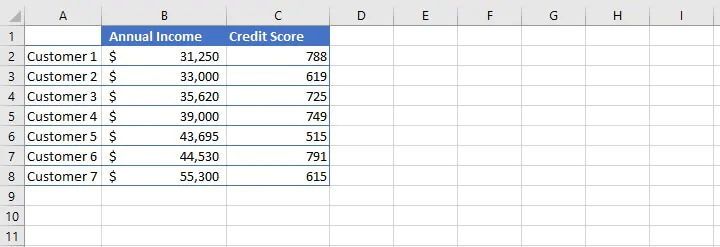The AND formula would be written as follows:

``=AND(B2>=35000,C2>=650)``

Customer 1 would not qualify for a loan since they failed logical statement one. The output in cell D2 would be FALSE. The result of copying the formula to cells D3 to D8 is shown below.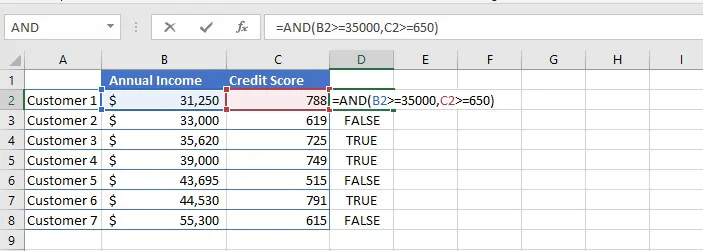In the image above, only the rows which met both qualifying criteria would have a value of TRUE displayed in column D.

## Use with text values

The AND function may also be used to evaluate whether a cell matches a specified text value. Text values must be enclosed in double quotation marks.

### Example 2

The dataset below shows customers traveling to one of two destinations and their day of departure.

The formula in column D identifies those whose destination is Grenada, and who are traveling on Friday. The text “Grenada” and “Friday” are entered within double quotes. The text is not case sensitive.

``=AND(B2= “Grenada”,C2= “Friday”)``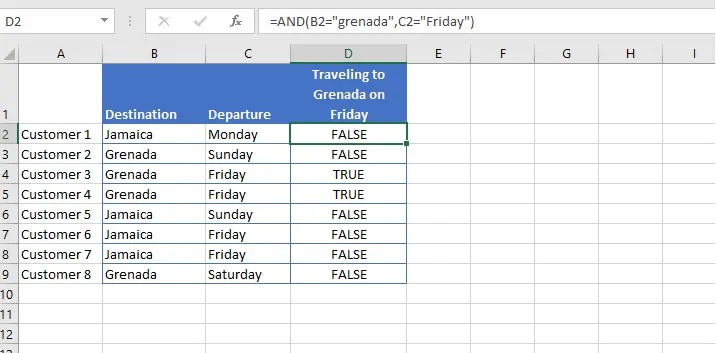Text which is not placed within double quotes will return a #NAME? Error.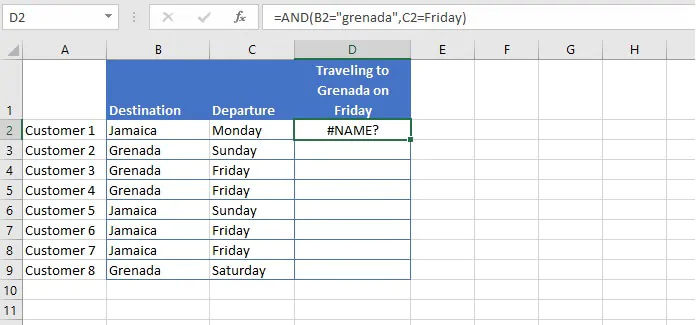## Use with IF

We can customize the output of the AND function, so that instead of returning a TRUE or FALSE result, Excel will display some other text, or perform a mathematical calculation that we specify. This is done by nesting the AND function into the IF function. A nested statement is one where one function is used as the argument of another function.

Let’s return to our bank loan example. Excel can return a value of “Approved” instead of TRUE, and “Not Approved” instead of FALSE by using the entire AND statement we used previously as the first argument in the IF function:

``AND(B2>=35000,C2>=650)``

The nested formula would read as follows:

``=IF(AND(B2>=35000,C2>=650), “Approved”, “Not Approved”)``

This simply means that Excel is evaluating the AND statement and the word “Approved” is the value_if_true of the IF function, whereas the words “Not Approved” is the value_if_false.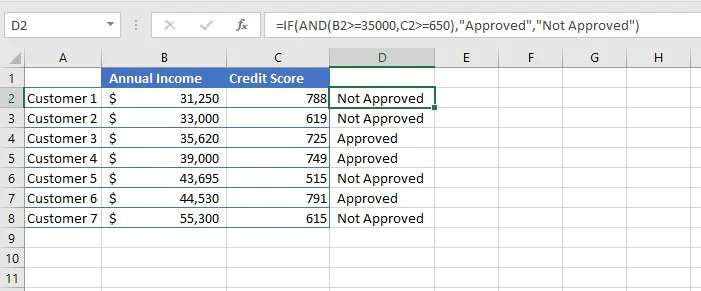As mentioned before, combining the IF and AND functions also allows Excel to return a mathematical calculation if the AND statement is true, thereby eliminating a number of intermediate steps.

In the bank loan scenario, we could have Excel calculate 20% of the applicant’s annual income as the loan amount for which they are approved.

``=IF(AND(B2>=35000,C2>=650),B2*0.2, “Not Approved”)``

This formula would both determine whether the applicant satisfies both conditions, and calculate the amount they are approved for while returning a value of “Not Approved” for those who failed one or both conditions.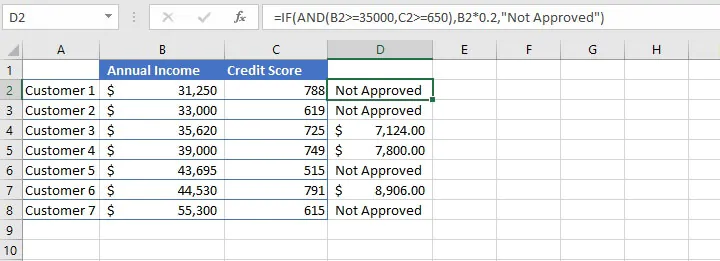## Use with OR

The AND function is similar to the OR function in some ways but is different in one very important way. While all stated criteria must be satisfied for the AND function to return a value of TRUE, the OR function will return a TRUE value if any of the stated criteria is satisfied.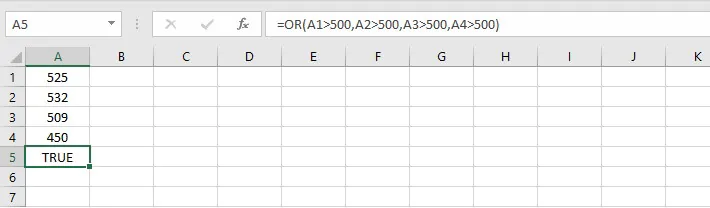The AND function can be nested with the OR function to come up with creative statements and solutions for common situations.

Reverting to the bank loan example, the bank may expand the qualification criteria by stating that applicants who do not meet the annual income requirement, but who are homeowners, may qualify for the loan, provided that their credit score is at least 650.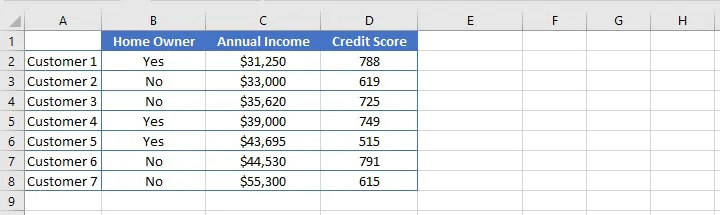A simplified way to represent these conditions would be:

 1. Homeowner OR Income > \$35,000 AND: 2. Credit score >= 650

Translated into Excel, the nested formula would be:

``=AND(OR(B2=“Yes”, C2>=35000), D2>=650)``

Nesting the OR statement inside the AND formula makes the OR formula the first argument of the AND formula. This allows for the flexibility of satisfying either homeowner or income status but maintains the condition that the applicant must also satisfy the minimum credit score.

### Nesting order of AND/OR functions

It is important to distinguish between nesting the OR function within the AND function and nesting the AND function within the OR function. Because of the different ways in which they work, it is useful to identify which formula is being placed as an argument of the other function.

For example, look at the following nested functions:

``=AND(OR(B2="Yes",C2>=35000),D2>=650)``
``=OR(AND(B2="Yes",C2>=35000),D2>=650)``

Column E below shows the result of the first formula, and column F shows the result of the second.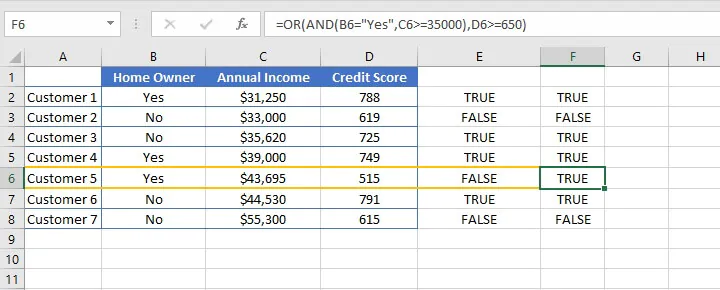In the case of the first formula, the applicant must either be a homeowner or be earning at least \$35,000. In either case, they must also have a credit score of at least 650.

In the case of the second formula, the applicant must either be a homeowner who is earning at least \$35,000 or must have a credit score of at least 650. This means an applicant who fails the credit score criteria but who is a homeowner earning \$35,000 or more would qualify, as seen in the case of Customer 5.

Of course, this may be desirable in some cases, but it is important to recognize the difference in how nesting the two functions works.

## Nesting IF/AND/OR

Finally, multiple functions may be nested within one formula. We can combine the IF, AND and, OR functions to calculate the amount each applicant qualifies for. For those who failed to qualify, we can type empty double quotes for the value_if_false to return a blank cell.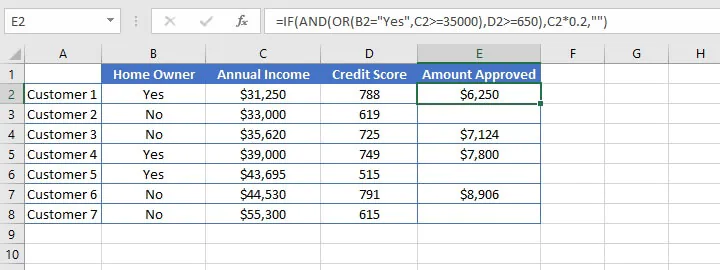## Summary

The Excel AND function is a useful tool that can be used to identify cells that satisfy multiple conditions, and it may be combined with other functions to maximize their potential as illustrated above. It can also enhance features such as sorting and filtering in Excel to maximize their potential.

Learn more Excel functions, formulas, and tips from the Microsoft Excel - Basic and Advanced course today. Or try our free Excel in an Hour course for some basic formula knowledge.

Start learning formulas, functions, and time-saving hacks today with this free course!#### Join the Excel conversation on Slack

Ask a question or join the conversation for all things Excel on our Slack channel.Claudia Buckley

Claudia is a project manager and business skills instructor at GoSkills. In her spare time, she reads mystery novels and does genealogy research.